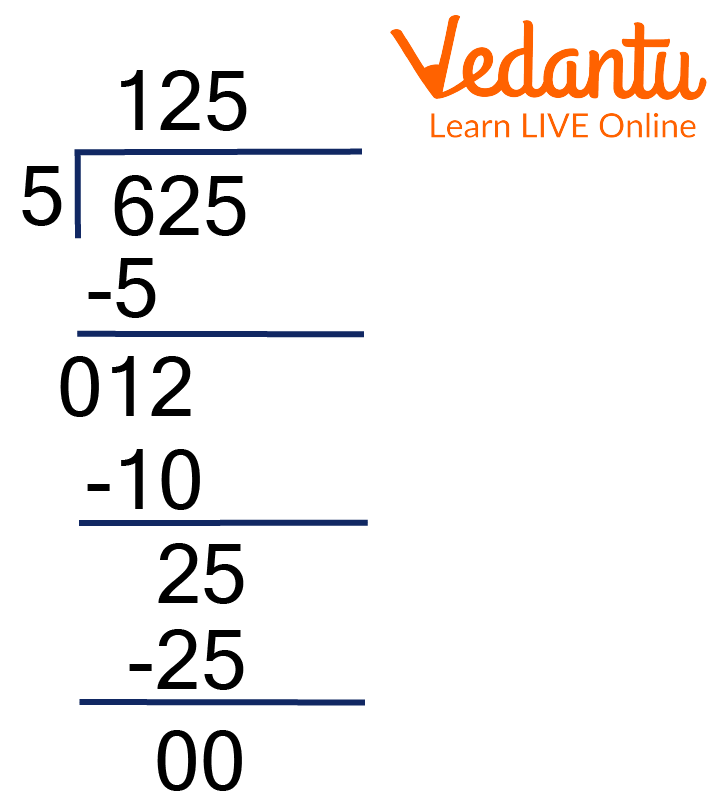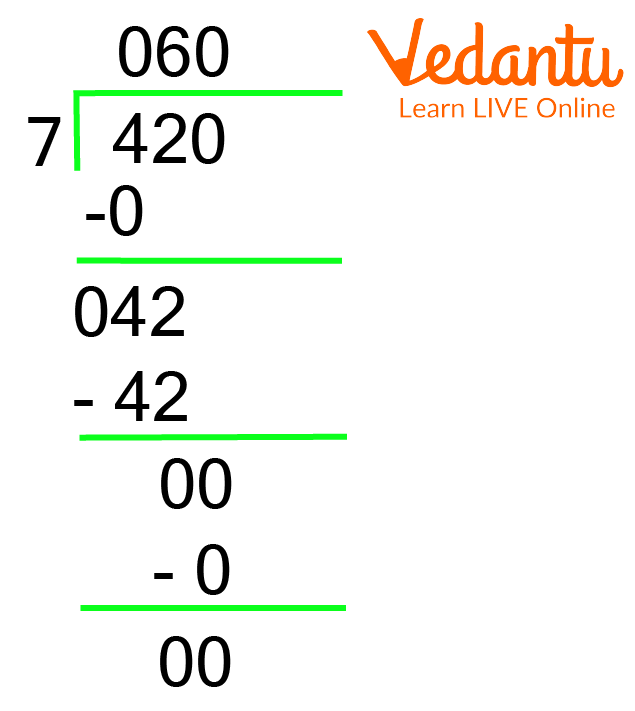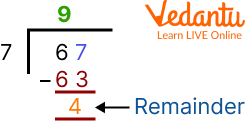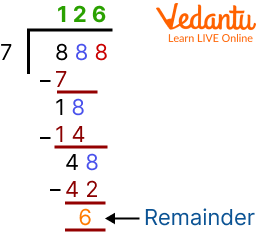Courses
Courses for Kids
Free study material
Free LIVE classes
More

# How to Find the Quotient and Remainder of a Number?LIVE
Join Vedantu’s FREE Mastercalss

## What is the Quotient of a Number?

Division can be defined as splitting large numbers into smaller groups having equal numbers each. The number of smaller groups obtained is called the quotient of a number. The quotient is the result obtained at the end of division like how sum, difference and product are for addition, subtraction and multiplication, respectively.Dividing Cake using Division

Division is one of the most important mathematical operations used during calculations to obtain equal parts of the given whole of something. So when you are required to cut a whole cake into 12 slices of equal sizes, as you have 12 friends to share it with, you’re applying division.

## What Should I Know to do Division?

If you have an idea of the other 3 mathematical operations listed below and a basic knowledge of divisibility you can master division too!

• Multiplication tables till 9

• Subtraction

• Divisibility rules till 9 (This is optional to know if the number would produce a remainder or not)

## What is Division?

Division is a process by which a large number is split into smaller groups each containing an equal number of parts in each group. It involves the multiplication and subtraction of some form to obtain the result known as the quotient. Another important aspect of division is the remainder which is the number that is left out after the process of division. Shown below is the symbol of division which is a line with dots placed above and below.Division Symbol

## How to Find the Quotient of a Given Perfectly Divisible Number?

For perfectly divisible numbers, we carry out the division till we obtain a remainder of 0 as illustrated below. We are to divide 625 by 5 as follows:Division of 625 by 5

Here, the quotient obtained is 125 and the remainder obtained is 0. The simple division is carried out till a remainder of 0 is obtained in the case of a perfectly divisible number.

## How to Find the Quotient and Remainder of a Given Number?

The above case illustrated is when the given number is perfectly divisible by the divisor. If the number given is not divisible by the divisor, a remainder is often obtained. To understand this case, we are going to divide 100 by 8 as follows:Division of 100 by 8

Here, the quotient obtained is 12 and the remainder obtained is 4. Simple division is carried out till a remainder obtained is lesser than the divisor in case of a number that is not divisible by the divisor.

## Solved Examples

1. Find the quotient and remainder obtained when 420 is divided by 7.

Ans: We carry out the division as follows:Division of 420 by 7

The quotient is found to be 60 and the remainder is found to be 0.

2. Divide 67 by 7.

Ans:Division of 67 by 7

The quotient is 9 and the remainder will be 4.

3. Divide 888 by 7.

Ans:Division of 888 by 7

The quotient is 126 and the remainder is 6.

## Practice Problems

1. Divide 506 by 7 and give the quotient and remainder.

Ans: Quotient - 72, remainder - 2.

2. Divide 782 by 5 and give the quotient and remainder.

Ans: Quotient - 156, remainder - 2.

3. Divide 932 by 4 and give the quotient and remainder.

Ans: Quotient - 233, remainder - 0.

4. Divide 327 by 2 and give the quotient and remainder.

Ans: Quotient - 163, remainder - 1.

5. Divide 144 by 3 and give the quotient and remainder.

Ans: Quotient - 48, remainder - 0.

## Summary

Division is a basic mathematical operation where a larger number is split into smaller numbers containing equal groups. The result obtained at the end of the division is known as the quotient. The number that is left out at the end of the process of division which cannot be divided any further is called the remainder.

If the larger number divided by the smaller number is perfectly divisible by it, then we only obtain a quotient and the remainder is usually 0. If the larger number divided by the smaller number is not divisible by it, then we obtain a quotient and the remainder is the number that is left out at the end.

Last updated date: 28th Sep 2023
Total views: 94.8k
Views today: 1.94k

## FAQs on How to Find the Quotient and Remainder of a Number?

1. What are the parts of division?

The process of division has 4 parts, namely divisor, dividend, quotient and remainder. Divisor is the number that is going to divide the given number into an equal number of parts. It is usually the smaller number.

Dividend is the number that is going to be divided into an equal number of parts. It is usually the larger number. Quotient is the result obtained at the end of the division, it is the number of parts that the dividend is divided into. Remainder is the parts left that cannot be divided further, it is usually lesser in value than the divisor.

2. What are the steps involved in division?

The steps to do division are listed below:

• Step 1 - Take the first digit of the dividend. Check if this digit is greater than or equal to the divisor.

• Step 2 - Then divide the first digit of the dividend by the divisor and write the answer on top and product below.

• Step 3 - Subtract the product result of step 2 from the digit and write the difference below and write down the next digit.

• Step 4 - Repeat the same process till the remainder is obtained to be 0 or lesser in value to the divisor.

3. What are the fundamentals of division?

The mathematical procedure of splitting a number into equal groups is called division. The dividend, divisor, quotient and remainder are the four basic components of a division.